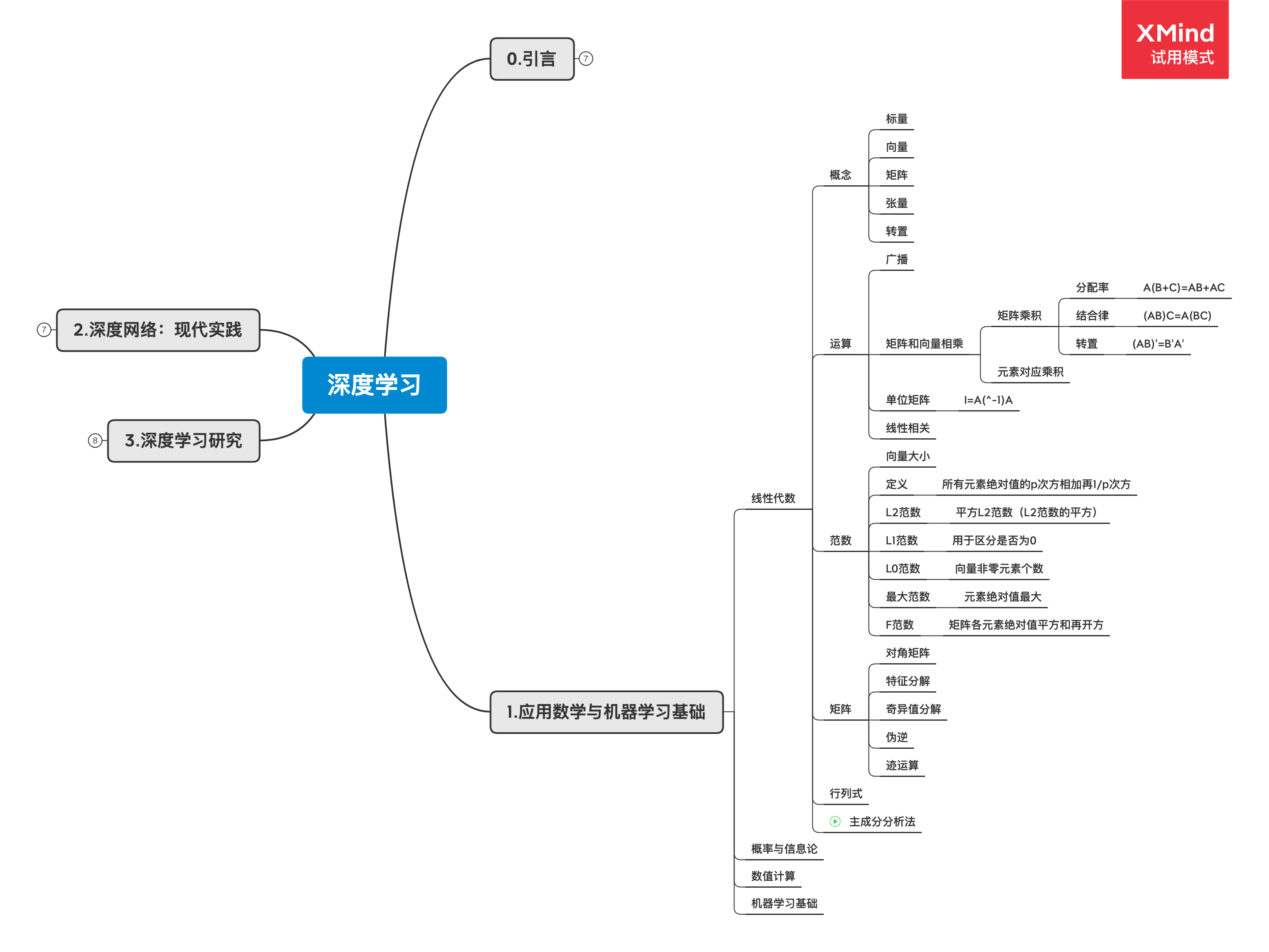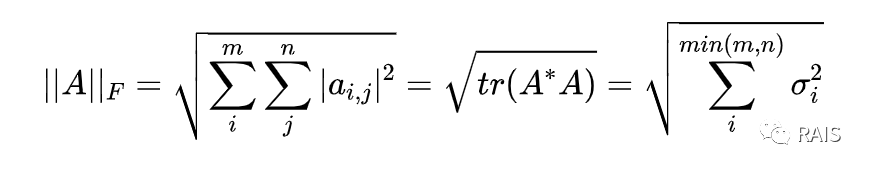# 深度学习中的线性代数

Posted by RAIS on 2020-03-26

## 深度学习中的线性代数### 易混基础概念

• 标量：单独一个数
• 向量：一行/列数
• 矩阵：二维数组
• 张量：一般指多维（0 维张量是标量，1 维张量是向量，2 维张量是矩阵）
• 转置：沿主对角线折叠

### 范数

#### 向量范数

$$形式上 L^p 范数:||x||_p=\left(\sum_i|x_i|^p\right)^{\frac{1}{p}}$$

$$\begin{cases} {f(x)=0 => x=0}\\ {f(x+y)\leq{f(x)+f(y)}}\\ \bigvee\alpha\in{R},f(ax)=|a|f(x) \end{cases}$$

• 当 p=2 时，$L^2=||x||_2$范数(可简化写成||x||)称为欧几里得范数，可以用来计算距离。但是我们看到这里有一个开方运算，因此为了去掉这个开方，我们平时用的有可能是范数的平方，这就会减少一次开方运算，在后面提到的损失函数中，范数和平方范数都提供了相同的优化目标，因为范数的平方计算起来也更简单，因此平方范数更常用。

• 当 p=1 时，$L^1$范数是向量各元素绝对值之和，在机器学习领域，对于区分 0 和非 0 来说，$L^1$范数很好用。

• 当 p=0 时，$L^0$实际上不是一个范数，但是大多数提到范数的地方都会提到$L^0$，$L^0$用来表示这个向量中有多少个非 0 元素，它是非常有用的，在机器学习中的正则化和稀疏编码中有广泛应用。在一个例子中是这么说的：判断用户名和密码是否正确，用户名和密码是两个向量，$L^0=0$时，则登录成功，$L^0=1$时，用户名和密码有一个错误，$L_0=2$时，用户名和密码都错误。

• 当 p 为无穷大时，范数也被称为无穷范数、最大范数。表示向量中元素绝对值中最大的，$L^\infty=max(|x_1|, |x_2|, …, |x_n|)$。

#### 矩阵范数• 对角矩阵
• 对称矩阵
• 单位向量
• 正交矩阵

### 特征分解

λ 为特征值组成的特征向量。

• $Av=λv$
• $A=Vdiag(λ)V^{-1}$
• 对称矩阵：$A=QΛQ^T$，Q 为 A 的特征向量，Λ 是对角矩阵。

### 线性代数的一些定义

• 对角矩阵：只有主对角线含有非零元素；
• 单位向量：具有单位范数的向量；
• 向量正交：如果两个向量都非零，则夹角 90 度；
• 标准正交：相互正交、$L^2$ 范数为 1；
• 正交矩阵：行向量和列向量分别标准正交；
• 特征分解：将矩阵分解为特征向量和特征值；
• 特征值和特征向量：Ax=λx 中的 λ 和 x；
• 正定、半正定、负定：特征值都正、非负、都负。

### 总结